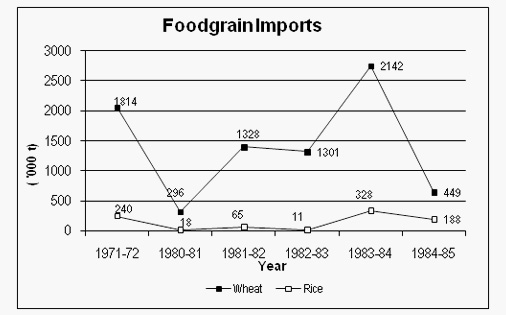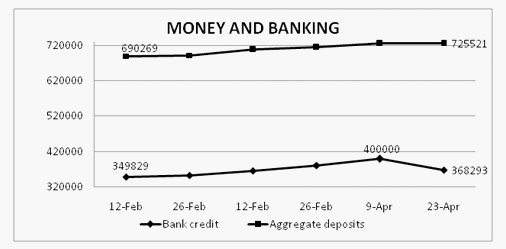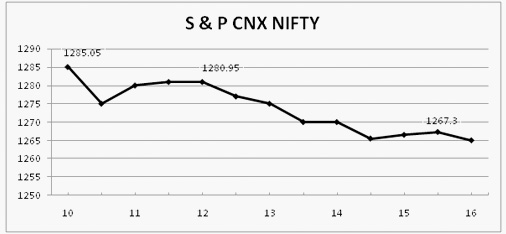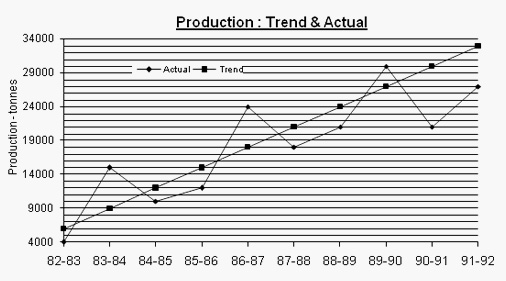# Line Graph Practice Questions: Level 02

DIRECTIONS for question 1 to 3: Refer to the following graph and answer the line graph problems that follow.
The total values of foodgrains (rice and wheat) imported during these years are given below:1971-72 : Rs. 123 crore
1980-81 : Rs. 80 crore
1981-82 : Rs. 314 crore
1982-83 : Rs. 295 crore
1983-84 : Rs. 587 crore
1984-85 : Rs. 158 crore
1. Wheat formed what percent of the volume of total imports of food grains from 1980 – 85?
1. 75%
2. 66%
3. 90%
4. 95%
5. 87%
2. If the import price of wheat was Rs. 2,400 per tonne in 1983-84, then what was the import price of rice per tonne during that year (in Rs.)?
1. 3,200
2. 2,225
3. 2,850
4. 1,800
5. Can't say
3. In which year was the ratio of rice to wheat imports the highest?
1. 1971 – 72
2. 1980 – 81
3. 1983 – 84
4. 1984 – 85
5. 1981 – 82
DIRECTIONS for questions 4– 6: Refer to the line graph below and answer the questions that follow:4. Given below are three statements. Select from the choices given, the FALSE statement(s).
I. The growth rate in aggregate deposits has been more than the growth in bank credit over the given period.
II. The percentage of bank credit to aggregate deposits has declined over the given period.
III. The growth of bank credit follows the same trend as the growth in aggregate deposits.
1. Only I
2. I and II
3. Only III
4. I, II & III
5. II and III
5. If CRR (percentage of deposits to be kept as reserves by banks) is 12%, then what is the amount of money which is lying unproductive in banks as on April 23?
1. Rs 2,701,265 crore
2. Rs 3,001,685 crore
3. Rs 2,803,733 crore
4. Rs 270,175 crore
5. Rs. 87,062 crore
6. If the trend of the period Apr 9-Apr 23 continues, what would be the bank credit as on May 7?
1. 320
2. 330
3. 338
4. 345
5. 450
DIRECTIONS for questions 7 and 8: Refer to the line graph below and answer the questions that follow.
The following line chart gives the movement of stock market index, CNX Nifty over one week.7. If I had invested Rs. 100 in S&P CNX Nifty stocks at the beginning of the time period, what would be its nearest value at the end of the period?
1. Rs. 99.50
2. Rs. 98.50
3. Rs. 97.50
4. Rs. 96.50
5. Rs. 100.50
8. Price volatility (PV) is defined as: [(Highest - Lowest Price) / Average Price]. The PV of S&P CNX Nifty is closest to
1. 0.10
2. 0.001
3. 0.014
4. 1.0
5. None of these
DIRECTIONS for questions 9-10: Refer to the line graph below and answer the questions that follow.
Following questions are based on the Line Graph given below.9. What has been the maximum deviation of actual from the trend?
1. –20%
2. +33%
3. +66%
4. –40%
5. –30%
10. How many times has the actual production shown a more than 50 % increase over the previous year?
1. Once
2. Twice
3. Thrice
4. Never
5. Four times